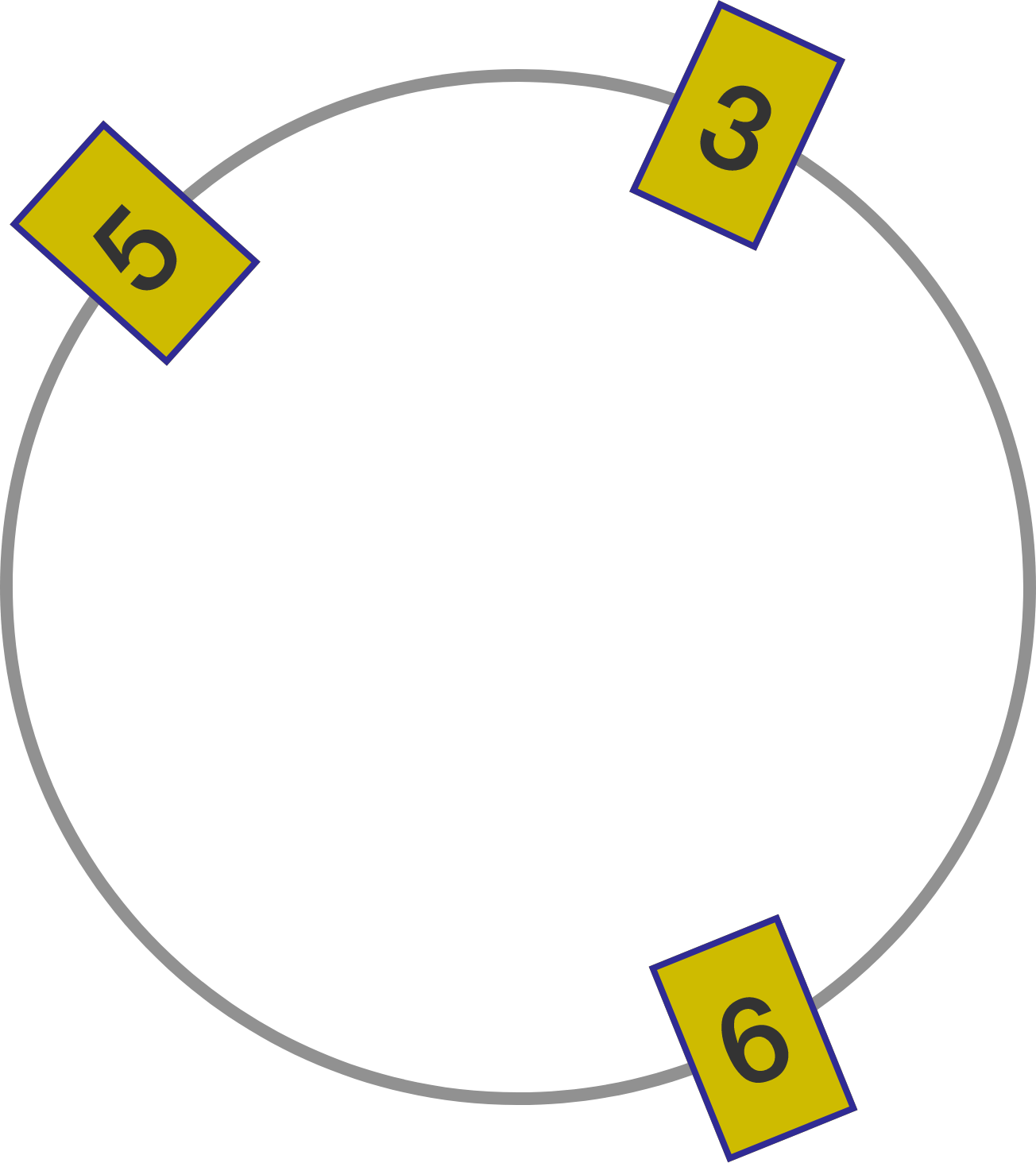# Digits in a circleSuppose we place $K$ distinct digits around a circle such that each pair of adjacent digits (read either clockwise or counterclockwise) forms a number that's divisible by $7.$ What's the maximum possible value of $K?$

For example, the diagram to the right illustrates a solution for $K=3.$ Note that 35, 56, and 63 are all multiples of 7.

Note: Not all pairs of digits need to be read in the same direction. Some pairs may be read clockwise and others may be read counterclockwise.

×